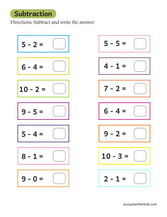This page features addition worksheets subtraction 1st grade. These are free highly illustrated test sheets which teachers and parents can use to review first grade subtraction at home or in the classroom. Simple click on the buttons below to preview and start the print out process. These worksheets will get students hooked based on their outlook and constitution of graphics.

### SubtractionBasic subtraction to ten worksheet

If you are looking for a fun way to teach children subtraction, you can use a number of games or activities on this website. A bowling game can be a great way to introduce the concept of subtraction and encourage your child to participate in this fun activity. Once your child has internalized the concept, they can use this game to improve their math skills. One LEGO subtraction game requires children to roll a die and subtract the pins from the total score. Another game involves racing LEGO bricks and the first one to reach zero wins. You can also use locks as subtraction objects. Amazon has a variety of locks in different colors that kids can use for years.

## Worksheets

Subtraction worksheets for first grade are useful for teaching students how to subtract single-digit numbers. Students learn how to solve problems that require them to remove the missing number or add it to a number they already have. They also learn how to subtract 2-digit numbers without taking credit, a skill they must master in second grade. Students can click on the links below to view and print the worksheets. Each worksheet includes an interactive preview that students can use to check for correct answers.

## Games

Games for subtraction in 1st grade can be played with blocks or other objects that represent numbers. One activity is a 3-D ten frame made of holes in a shoebox. Another game is to knock over ping pong balls or Lego blocks to see how many are left. Another fun activity is reenacting a story. Stacking cards with numbers is another fun activity. Racing to the finish line can help students learn about place value, and some games require children to use and practice flashcards.

## Activities

For your first grade subtraction students, there are many ways to engage them in the process. One fun way is to have students act out a math story using a tens frame, number paths, or other manipulatives. Students act out a subtraction story using objects and share the answer with the group. They can then repeat the activity with their classmates. These activities are ideal for practicing math skills while reinforcing the importance of collaboration.

## Techniques

Subtraction in the first class requires the use of a variety of methods. One method is to count up from the subtraction to the whole number. This works best for numbers with up to ten digits. This method plays with double facts and reinforces counting by tens. Here are some techniques to help your child grasp the concept of multi-digit subtraction in first grade.

## Resources

If you are looking for 1st grade subtraction resources, you have come to the right place! Resources for first grade subtraction include word games, number coloring activities, and pizza making. You can also find resources for subtraction beyond first grade if you are looking for ways to support your students' learning. Below, we have compiled some resources for subtraction strategies.